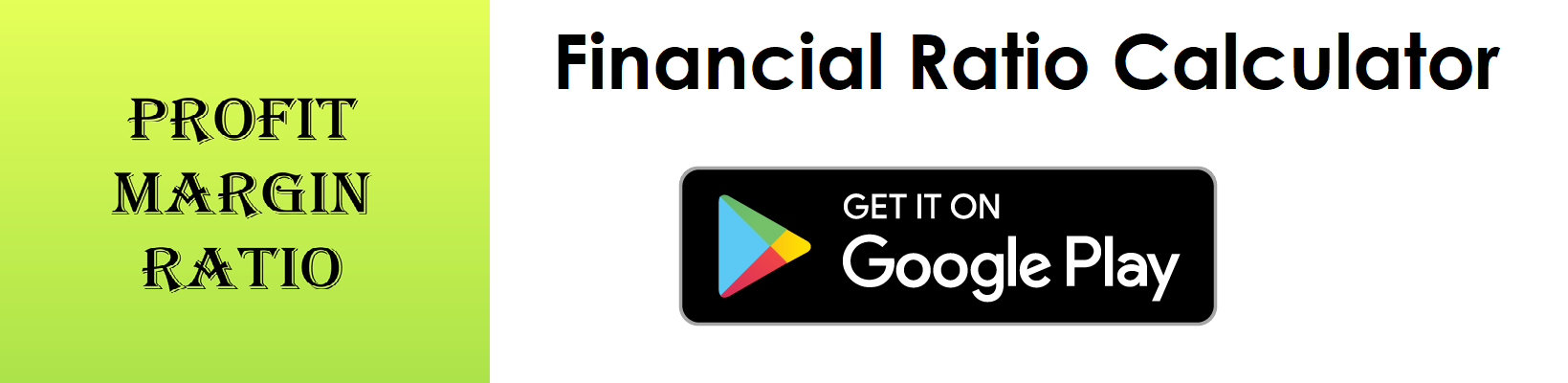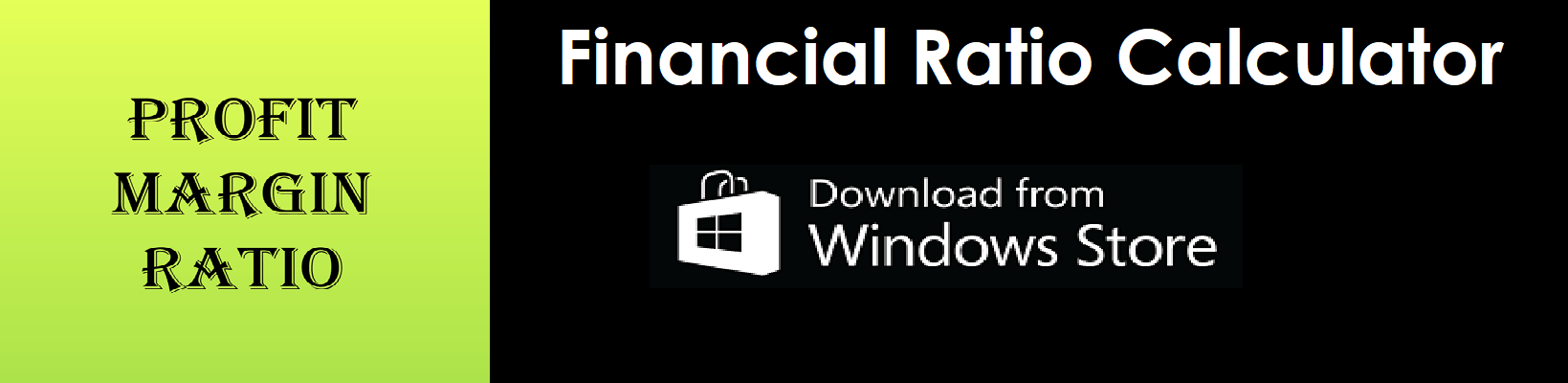# Return on Assets Ratio Calculator

Key in the Net Income Values from your Income Statement and Total Assets Value from your Balance Sheet to the respective fields given below and then click Calculate to get the desired result.

##### Return on Assets Ratio Calculator - Glossary:
Using our return on assets ratio calculator, helps to compare, measure, understand the overall health of the business.

##### Return on Assets:
Shows the proportion of net income to average total assets. In simple words, how efficiently a company can convert the money used to purchase assets into net income or profits.

##### How to calculate?
Formula: Return on Assets (ROA) = Net Income / Total Assets.
The values for net income can be obtained from income statement and total assets value from the balance sheet.

##### Net Income:
Profit left over after deducting all its expenses (cost of goods, operational expenses, interest cost, depreciation cost, taxes and if any dividend paid).

##### Total Assets:
Total assets are the sum of all current and noncurrent assets that a company owns.

##### Example:
Return on assets ratio for a company with net income of \$60,000 and total assets of\$300,000 is 20%. It means, every dollar that was invested in assets during the year produced \$0.20 of net income.

# Download our Mobile Apps - today.